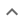•# Other Factorizations

We think you wrote:

###This solution deals with other factorizations.

Solution found-5*(x^6-8)

## Step by Step Solution### Reformatting the input :

(1): "x6"   was replaced by   "x^6".

## Step  1  :

#### Equation at the end of step  1  :

  40 -  5x6


## Step  3  :

#### Pulling out like terms :

Pull out like factors :

40 - 5x6  =   -5 • (x6 - 8)

#### Trying to factor as a Difference of Squares :

Factoring:  x6 - 8

Theory : A difference of two perfect squares,  A2 - B2  can be factored into  (A+B) • (A-B)

Proof :  (A+B) • (A-B) =
A2 - AB + BA - B2 =
A2 - AB + AB - B2 =
A2 - B2

Note :  AB = BA is the commutative property of multiplication.

Note :  - AB + AB equals zero and is therefore eliminated from the expression.

Check : 8 is not a square !!

Ruling : Binomial can not be factored as the difference of two perfect squares.

### Polynomial Roots Calculator :

Find roots (zeroes) of :       F(x) = x6 - 8
Polynomial Roots Calculator is a set of methods aimed at finding values of  x  for which   F(x)=0

Rational Roots Test is one of the above mentioned tools. It would only find Rational Roots that is numbers  x  which can be expressed as the quotient of two integers

The Rational Root Theorem states that if a polynomial zeroes for a rational number  P/Q   then  P  is a factor of the Trailing Constant and  Q  is a factor of the Leading Coefficient

In this case, the Leading Coefficient is  1  and the Trailing Constant is  -8.

The factor(s) are:

of the Leading Coefficient :  1

of the Trailing Constant :  1 ,2 ,4 ,8

Let us test ....

P  Q  P/Q  F(P/Q)   Divisor
-1     1      -1.00      -7.00
-2     1      -2.00      56.00
-4     1      -4.00      4088.00
-8     1      -8.00     262136.00
1     1      1.00      -7.00
2     1      2.00      56.00
4     1      4.00      4088.00
8     1      8.00     262136.00

Polynomial Roots Calculator found no rational roots

## Final result :

  -5 • (x6 - 8)